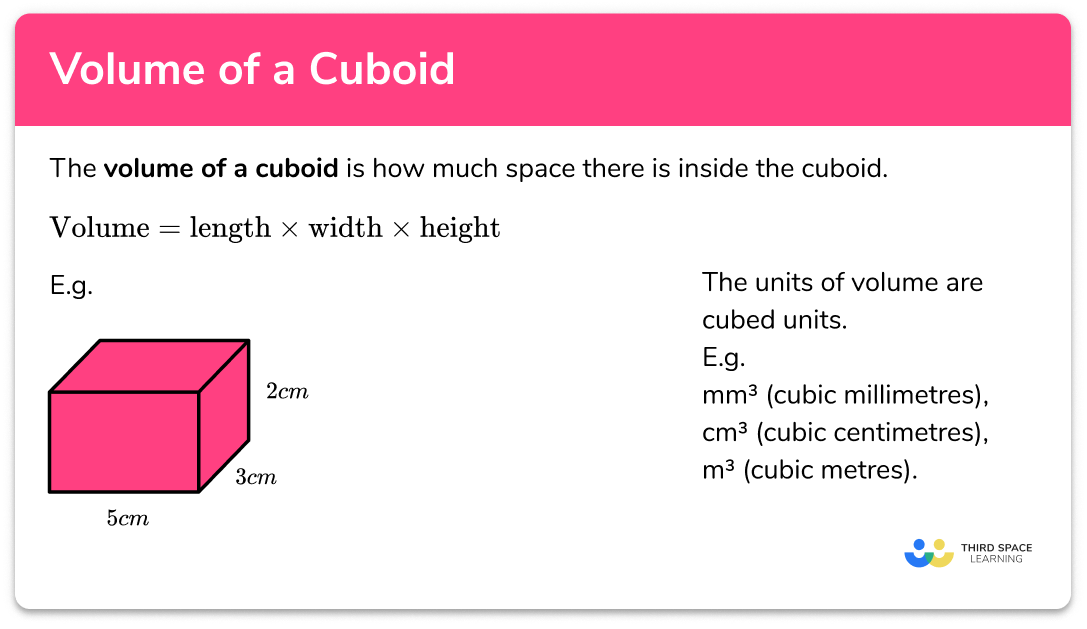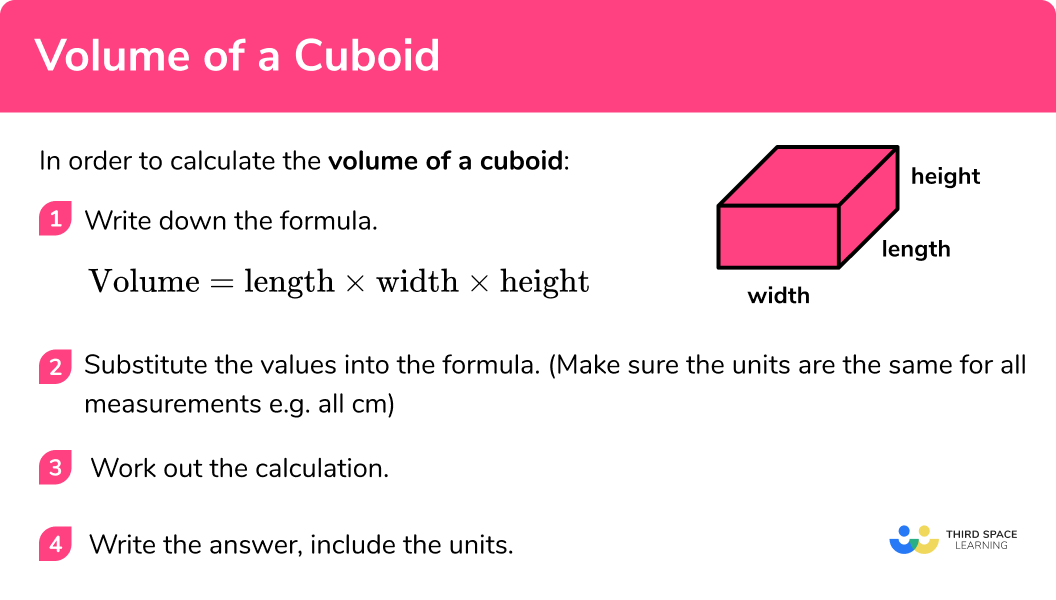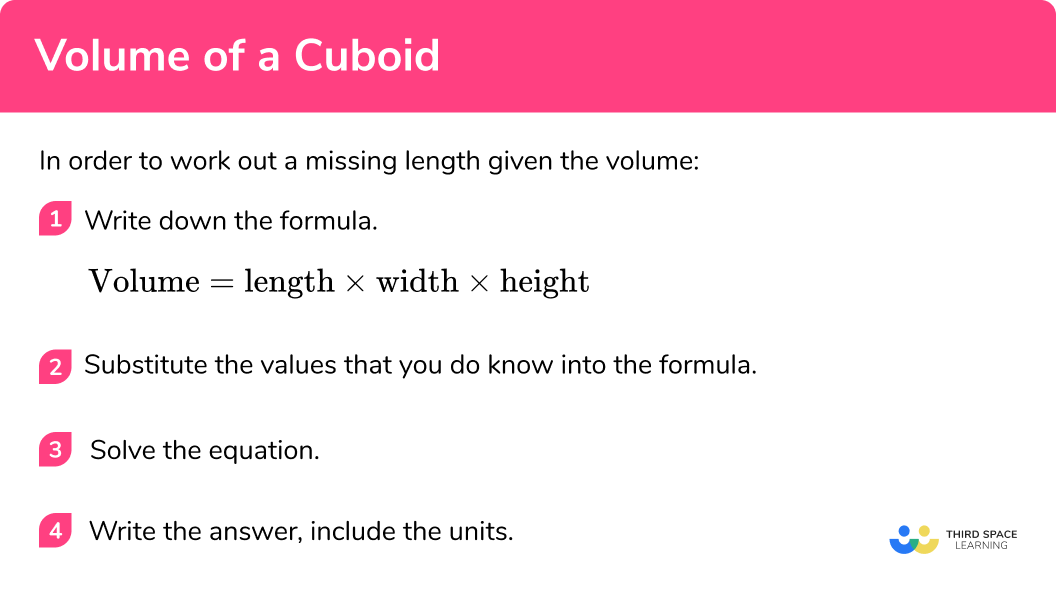# Volume Of A Cuboid

Here we will learn about the volume of a cuboid, including how to calculate the volume of a cuboid and how to find missing lengths of a cuboid given its volume.

There are also volume and surface area of a cuboid worksheets based on Edexcel, AQA and OCR exam questions, along with further guidance on where to go next if you’re still stuck.

## What is volume of a cuboid?

The volume of a cuboid is the amount of space there is within a cuboid.

Cuboids are 3 dimensional shapes with 6 rectangular faces.

The formula for the volume of a cuboid is:

Volume = length \times width \times height

Volume is measured in cubic units, e.g. mm^3, cm^3 or m^3.

This cuboid is made from 24 unit cubes.

Its volume is

\begin{aligned} &Volume = length \times width \times height \\ &Volume = 2 \times 4 \times3 \\ &Volume = 24 \; cm^3 \end{aligned}

The units of volume are cubed units

E.g. mm^3 (cubic millimetres), cm^3 (cubic centimetres), m^3 (cubic metres) .

### What is volume of a cuboid?## How to calculate the volume of a cuboid

In order to calculate the volume of a cuboid:

1. Write down the formula.

Volume = length \times width \times height

2Substitute the values into the formula.

Make sure the units are the same for all measurements

3Substitute the values into the formula.

4Write the answer, include the units.

### How to calculate the volume of a cuboid## Volume of a cuboid examples

### Example 1: volume of a cuboid

Work out the volume of the cuboid:

1. Write down the formula.

Volume = length \times width \times height

2Substitute the values into the formula.

Here the length is 10 \; cm , the width is 2 \; cm and the height is 5 \; cm .

Volume = 2 \times 10 \times 5

3Work out the calculation.

\begin{aligned} &Volume = 2 \times 10 \times 5 \\\\ &Volume = 100 \end{aligned}

4Write the answer, include the units.

The measurements are in cm therefore the volume will be in cm^3.

Volume = 100cm^3

### Example 2: volume of a cube

Work out the volume of this cube:

Write down the formula.

Substitute the values into the formula.

Work out the calculation.

Write the answer, include the units.

### Example 3: volume of a cuboid (different units)

Work out the volume of this cuboid:

Write down the formula.

Substitute the values into the formula.

Work out the calculation.

Write the answer, include the units.

## How to work out a missing length given the volume

Sometimes we might know the volume and some of the measurements of a cuboid and we might want to work out the other measurements. We can do this by substituting the values that we know into the formula for the volume of a cuboid and solving the equation that is formed.

1. Write down the formula.

Volume = length \times width \times height

2Substitute the values that you do know into the formula.

Make sure the units are the same for all measurements

3Solve the equation.

4Write the answer, include the units.

### How to work out a missing length given the volume## Missing length given the volume examples

### Example 4: find the width of a cuboid given the volume

The volume of the cuboid is 56 \; cm^3 . Work out the width of the cuboid.

Write down the formula.

Substitute the values that you do know into the formula.

Solve the equation.

Write the answer, include the units.

### Example 5: find the length of a cuboid given the volume

The cuboid below has a square base. The height of the cuboid is 8 \; m and the volume of the cuboid is 32 \; m^3 . Find the length of the cuboid.

Write down the formula.

Substitute the values that you do know into the formula.

Solve the equation.

Write the answer, include the units.

### Example 6: dimensions of a cube given the volume

Work out the dimensions of a cube which has a volume of 64 \; cm^3

Write down the formula.

Substitute the values that you do know into the formula.

Solve the equation.

Write the answer, include the units.

### Common misconceptions

• Missing/incorrect units

You should always include units in your answer. Volume is measured in units cubed (e.g. mm^3, cm^3, m^3 etc)

• Calculating with different units

You need to make sure all measurements are in the same units before calculating volume.

E.g. you can’t have some in cm and some in m

• Dividing by three rather than cube rooting

If you are given the volume of a cube and you need to find the side length, remember the inverse of cubed is cube root, not divide by 3.

E.g. if the volume of a cube is 8cm^3 , the side length is

\sqrt{8}=2 \; \mathrm{cm} (not 8\div3 )

Volume of a cuboid is part of our series of lessons to support revision on cuboid. You may find it helpful to start with the main cuboid lesson for a summary of what to expect, or use the step by step guides below for further detail on individual topics. Other lessons in this series include:

### Practice volume of a cuboid questions

1. Work out the volume of the cuboid144 \; \mathrm{cm}^{2}12 \; \mathrm{cm}^{3}144 \; \mathrm{cm}^{3}36 \; \mathrm{cm}^{3}\begin{aligned} \text{Volume }&= \text{ length }\times \text{ width }\times \text{ height }\\\\ \text{Volume }&= 12 \times 3 \times 4\\\\ \text{Volume }&=144 \; \mathrm{cm}^{3} \end{aligned}

2. Work out the volume of the cube64 \; \mathrm{m}^{3}512 \;\mathrm{m}^{3}8 \;\mathrm{m}^{3}24 \; \mathrm{m}^{3}\begin{aligned} \text{Volume }&= \text{ length }\times \text{ width }\times \text{ height }\\\\ \text{Volume }&= 8 \times 8 \times 8\\\\ \text{Volume }&=512 \; \mathrm{m}^{3} \end{aligned}

3. Work out the volume of this cuboid1748 \; \mathrm{cm}^{3}174.8 \; \mathrm{m}^{3}17.48 \; \mathrm{m}^{3}17.48 \; \mathrm{cm}^{3}Here there are measurements in cm and m so we must make the units the same before calculating the volume.

We can convert 380 \; cm to 3.8 \;m.

\begin{aligned} \text{Volume }&= \text{ length }\times \text{ width }\times \text{ height }\\\\ \text{Volume }&= 2.3 \times 2 \times 3.8\\\\ \text{Volume }&=17.48 \; \mathrm{m}^{3} \end{aligned}

Since the measurements we used were in metres, the volume will be in cubic metres.

4. The volume of this cuboid is 600 \; cm^{3} .

Work out the height of the cuboid.3 \; cm24 \; cm200 \; cm75 \; cm\begin{aligned} \text{Volume }&= \text{ length }\times \text{ width }\times \text{ height }\\\\ 600&= 25 \times 8 \times h\\\\ 600&=200h\\\\ h&=3\; \mathrm{cm} \end{aligned}

5. The base of this cuboid is a square. The volume of the cuboid is 450\; cm^{3} .

Work out the height of the cuboid.45 \; cm4.5 \; cm2.1 \; cm6.7 \; cmSince the base of the cuboid is a square, the length and the width are both 10 \; cm.

\begin{aligned} \text{Volume }&= \text{ length }\times \text{ width }\times \text{ height }\\\\ 450&= 25 \times 10 \times h\\\\ 450&=100h\\\\ h&=4.5 \; \mathrm{cm} \end{aligned}

6. The volume of this cube is 343 \; cm^{3} .

What is the length of the cube?7 \; cm114.3 \; cm18.5 \; cm57.2 \; cm\begin{aligned} \text{Volume }&= \text{ length }\times \text{ width }\times \text{ height }\\\\ 343&=l \times w \times h \end{aligned}

Since this is a cube we know that the length, width and height are all equal. Therefore we are looking for a number that, when multiplied by itself 3 times, makes 343. We need the cube root of 343.

\sqrt{343}=7

The length, width and height of the cube are all 7 \;cm.

### Volume of a cuboid GCSE questions

1. Work out the volume of this cuboid. Give your answer in cm^3.(3 marks)

0.9 \; m = 90 \;cm

(1)

\text{Volume }=90 \times 40 \times 20

(1)

\text{Volume }=72000 \; \mathrm{cm}^{3}

(1)

2. A paddling pool is in the shape of a cuboid.(a) Work out the volume of the paddling pool.

(b) Sam wants to fill the pool so that it is \frac{5}{6} full.

Water flows out of her hose pipe and into the pool at a rate of 20 litres per minute.

Given that 1\;\mathrm{m}^{3}=1000 \;\mathrm{l} , calculate the length of time it would take Sam to fill the pool so that it is \frac{5}{6} full. Give your answer in hours.

(6 marks)

(a)
\text{Volume }=4 \times 1.8 \times 0.6

(1)

\text{Volume }=4.32 \; \mathrm{m}^{3}

(1)

(b)

4.32 \times 1000=4320 \; \mathrm{l}

(1)

\frac{5}{6} \text{ of } 4320 = 3600 \; \mathrm{l}

(1)

3600 \div 20=180 \text{ mins}

(1)

180 \; \text{ mins} = 3 \; \text{ hours}​

(1)

3. A carton of orange juice is shown below. The carton is completely full. The orange juice is poured into another container, as shown below.What will the height of the orange juice in the container be?

(5 marks)

\text{Volume of orange juice: }6 \times 10 \times 30

(1)

\text{Volume of orange juice: }1800 \; \mathrm{cm}^{3}

(1)

\begin{aligned} \text{Volume }&= \text{ length }\times \text{ width }\times \text{ height }\\\\ 1800&=40 \times 9 \times h \end{aligned}

(1)

1800 = 360 \;h

(1)

h = 5 \; cm

(1)

## Learning checklist

You have now learned how to:

• Know and apply the formula to calculate the volume of cuboids
• Use the properties of faces, surfaces, edges and vertices of cubes and cuboids to solve problems in 3-D

## Still stuck?

Prepare your KS4 students for maths GCSEs success with Third Space Learning. Weekly online one to one GCSE maths revision lessons delivered by expert maths tutors.

Find out more about our GCSE maths tuition programme.